# E-PolyLearning

 6. In continuous distillation, the internal reflux ratio (Ri) and the external reflux ratio (Re) are related as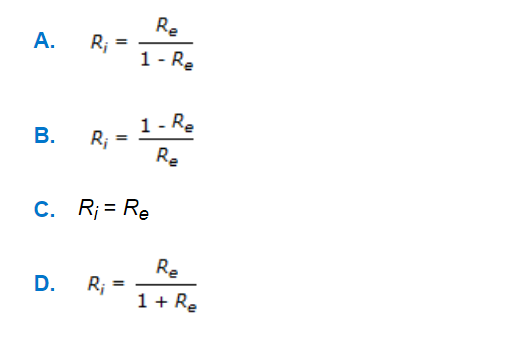a. A b. B c. C d. D

 7. The following equation is for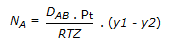a. steady state equimolar counter diffusion b. Fick's first law of diffusion c. steady state diffusion for stagnant case d. liquid M.T.C. by penetration theory
 8. The following equation is for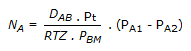a. Fick's second law of diffusion. b. stready state diffusion for stagnant case. c. liquid M.T.C. by Higbie penetration theory. d. none of these.
 9. The following plot gives the saturated humidity (He) versus temperature (T).Line joining (H1, T1) and (H2, T2) is the constant enthalpy line. Choose the correct one from among the alternatives A, B, C and D.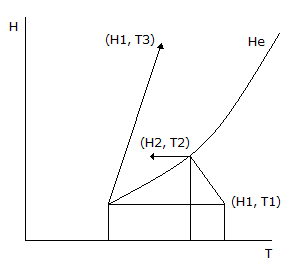a. T1-Dew point temp; T2-Dry bulb temp; T3-Wet bulb temp. b. T1-Dew point temp; T2-Wet bulb temp; T3-Dry bulb temp. c. T1-Wet bulb temp; T2-Dry bulb temp; T3-Dew point temp. d. T1-Dry bulb temp; T2-Wet bulb temp; T3-Dew point temp.
 10. Which of the following liquid temperature (T) Vs. vapor-gas mixture enthalpy (H) diagram is valid for humidification operation?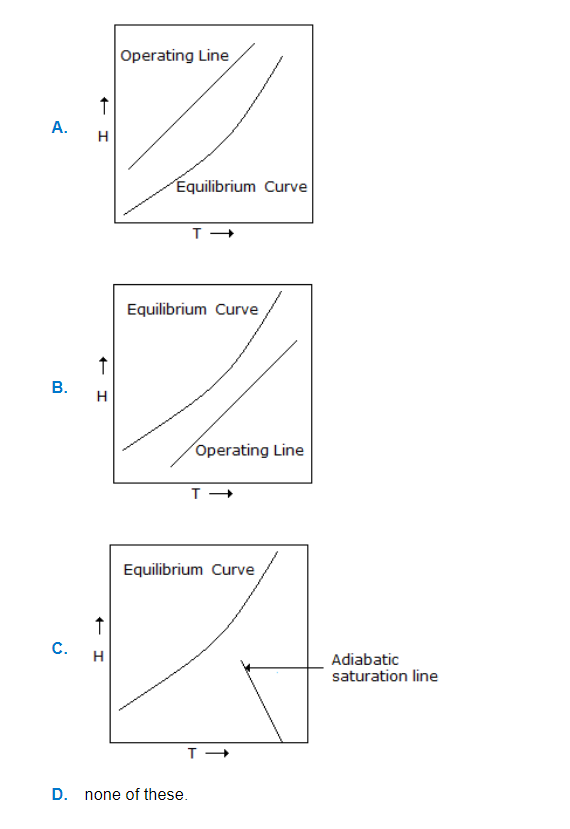a. A b. B c. C d. D